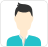Find the best tutors and institutes for Class 10 Tuition

Find Best Class 10 Tuition

Please select a Category.

Please select a Locality.

No matching category found.

No matching Locality found.

Outside India?

Search for topics

Exercise 6.2

In Fig. 6.17, (i) and (ii), DE || BC. Find EC in (i) and AD in (ii).(i)Let EC = x cm

It is given that DE || BC.

By using basic proportionality theorem, we obtain(ii) Let AD = cm

It is given that DE || BC.

By using basic proportionality theorem, we obtainTasneem

E and F are points on the sides PQ and PR respectively of a triangle PQR. For each of the following cases, state whether EF || QR :

(i) PE = 3.9 cm, EQ = 3 cm, PF = 3.6 cm and FR = 2.4 cm

(ii) PE = 4 cm, QE = 4.5 cm, PF = 8 cm and RF = 9 cm

(iii) PQ = 1.28 cm, PR = 2.56 cm, PE = 0.18 cm and PF = 0.36 cm(i)Given that, PE = 3.9 cm, EQ = 3 cm, PF = 3.6 cm, FR = 2.4 cm

Therefore, EF is not parallel to QR

(ii)PE = 4 cm, QE = 4.5 cm, PF = 8 cm, RF = 9 cm

therefore, EF is parallel to QR.PQ = 1.28 cm, PR = 2.56 cm, PE = 0.18 cm, PF = 0.36 cm

Hence

Therefore, EF is parallel to QR.Tasneem

In Fig., DE || OQ and DF || OR. Show that EF || QR.In Δ POQ, DE || OQ

(basic proportionality theorem)From (i) and (ii) we get,

(converse of BPT)Tasneem

In Fig., A, B and C are points on OP, OQ and OR respectively such that AB || PQ and AC || PR. Show that BC || QR.In Δ POQ, AB || PQFrom (i) and (ii) we getTasneem

Using Theorem 6.2, prove that the line joining the mid-points of any two sides of a triangle is parallel to the third side. (Recall that you have done it in Class IX).Consider the given figure in which PQ is a line segment joining the mid-points P and Q of line AB and AC respectively.

i.e., AP = PB and AQ = QC

It can be observed that   and

Hence, by using basic proportionality theorem, we obtainTasneem

ABCD is a trapezium in which AB || DC and its diagonals intersect each other at the point O. Show thatDraw a line EF through point O, such that

By using basic proportionality theorem, we obtain

In

So, by using basic proportionality theorem, we obtainTasneem

The diagonals of a quadrilateral ABCD intersect each other at the point O such that ⋅ Show that ABCD is a trapezium.

Consider the following figureDraw a line OE || ABIn ΔABD,

By using basic proportionality theorem, we obtain

⇒ EO || DC [By the converse of basic proportionality theorem]

⇒ AB || OE || DC

⇒ AB || CD

∴ ABCD is a trapezium.Tasneem

How helpful was it?

How can we Improve it?

Please tell us how it changed your life *

Please enter your question below and we will send it to our tutor communities to answer it *

Please select a tag

Name *

Enter a valid name.

Email *

Enter a valid email.

Email or Mobile Number: *

Please enter your email or mobile number

Sorry, this phone number is not verified, Please login with your email Id.